# Test: Laplace Transform- 2

## 10 Questions MCQ Test Topicwise Question Bank for Electrical Engineering | Test: Laplace Transform- 2

Description
Attempt Test: Laplace Transform- 2 | 10 questions in 30 minutes | Mock test for GATE preparation | Free important questions MCQ to study Topicwise Question Bank for Electrical Engineering for GATE Exam | Download free PDF with solutions
QUESTION: 1

### Consider the function f(t) having Laplace transform,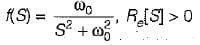the final value of f(t) would be

Solution: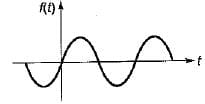L-1[F(S)] = sin ω0t
f(t) - sin ω0t
So, - 1 < f(∞) <1

QUESTION: 2

### The transfer function H(S) of a stable system is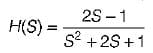the impulse response is

Solution: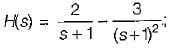System is stable
⇒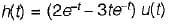QUESTION: 3

### If L[f(t)] =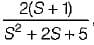then f(0+) and f(∞) are given by

Solution:
QUESTION: 4

Find the laplace transform of time function shown in figure.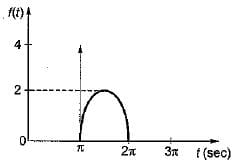Solution: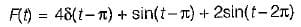Taking laplace,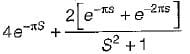QUESTION: 5

Consider the following signal: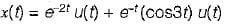the laplace transform of above system is

Solution:
QUESTION: 6

Consider a signal x(t) having laplace transform given by,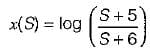The time domain signal x(t) is equal to

Solution:
QUESTION: 7

Given that: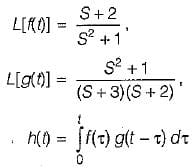then, L[h(t)] is

Solution:

Convolution in time domain is multiplication in s-domain.
∴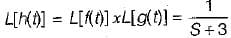QUESTION: 8

A causal LTI system is described by the difference equation, 2y[n] = αy[n- 2] - 2x[n] + βx[n - 1]. The system is stable only if,

Solution: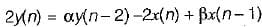Taking z-transform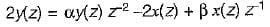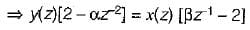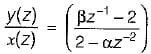For system to bs stable, β can be of any value.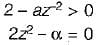⇒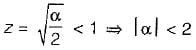For system to be stable all poles should be inside unity circle.

QUESTION: 9

Match List-I (Function in time domain) with List-II (Corresponding Laplace Transform F(S)) and select the correct answer using the codes given below the lists:
List-I
A.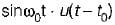B.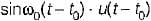C.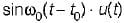D.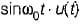List-II
1.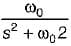2.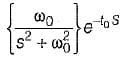3.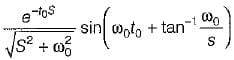4.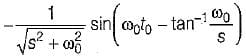Codes: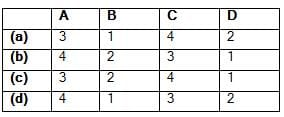Solution:
QUESTION: 10

The lapiace transform of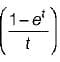Solution: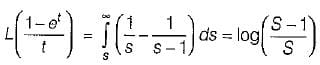Use Code STAYHOME200 and get INR 200 additional OFF Use Coupon Code